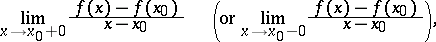# One-sided derivative

(diff) ← Older revision | Latest revision (diff) | Newer revision → (diff)
A generalization of the concept of a derivative, in which the ordinary limit is replaced by a one-sided limit. If the following limit exists for a functionof a real variable:then it is called the right (respectively, left) derivative ofat the point. If the one-sided derivatives are equal, then the function has an ordinary derivative at. See also Differential calculus.